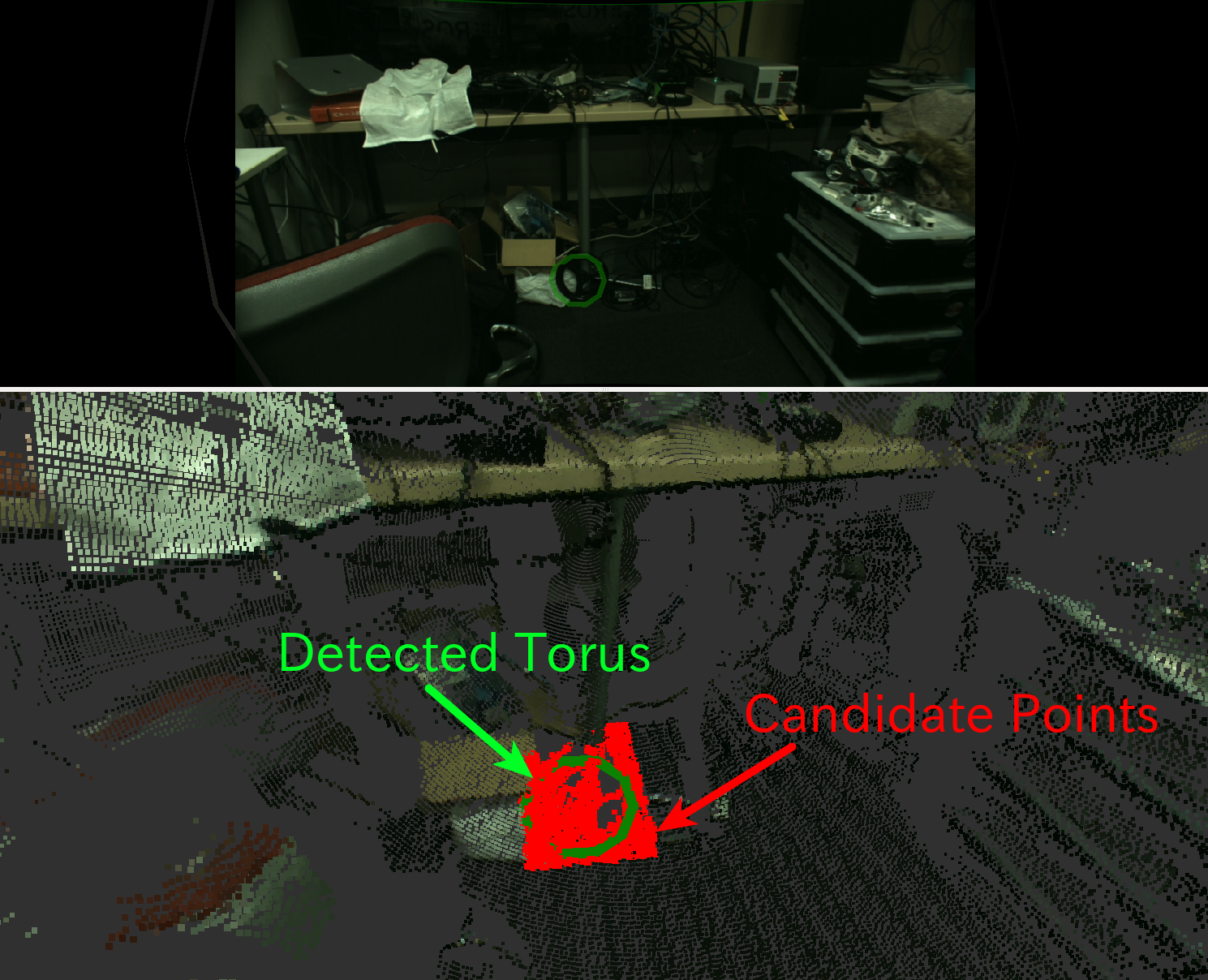# TorusFinder¶Find a torus out of pointcloud based on RANSAC with 3-D circle model.

## Subscribing Topic¶

• ~input (sensor_msgs/PointCloud2)

Input pointcloud. You may need to choose good candidates of pointcloud.

• ~input/polygon (geometry_msgs/PolygonStamped)

Input polygon. You can use this topic as well as ~input.

Vertices of the polygon will be used for detecting torus.

## Publishing Topic¶

• ~output (jsk_recognition_msgs/Torus)

Output of detection.

• ~output/inliers (pcl_msgs/PointIndices)

• ~output/coefficients (pcl_msgs/ModelCoefficients)

Inliers and coefficients which represents detection result.

• ~output/array (jsk_recognition_msgs/TorusArray)

Array of torus. It will be used for visualization.

• ~output/pose (geometry_msgs/PoseStamped)

Publish result of detection as geometry_msgs/PoseStamped

• ~output/with_failure (jsk_recognition_msgs/Torus)

• ~output/with_failure/array (jsk_recognition_msgs/TorusArray)

Output of detection with failure information.

• ~output/latest_time (std_msgs/Float32)

latest computation time

• ~output/average_time (std_msgs/Float32)

average computation time

## Parameters¶

• ~use_hint (Bool, default: False)

Whether to set hint axis which we need to search for a model perpendicular to.

• ~initial_axis_hint (Array of Float, default: [0, 0, 1])

The axis along which we need to search for a model perpendicular to.

This parameter is enabled only when ~use_hint is True.

• ~use_normal (Bool, default: False)

Whether to use normal field of input pointcloud.

Parameters below can be changed by dynamic_reconfigure.

• ~algorithm (String, default: RANSAC)

Type of sample consensus method to use.

Choose one from RANSAC, LMEDS, MSAC, RRANSAC, RMSAC, MLESAC and PROSAC.

• ~min_radius (Double, default: 0.1)

• ~max_radius (Double, default: 1.0)

Minimum and maximum radius of torus in meters.

• ~min_size (Integer, default: 10)

Minimum number of inliers.

• ~outlier_threshold (Double, default: 0.01)

Outlier distance threshold used in RANSAC in meters.

• ~max_iterations (Integer, default: 100)

Maximum number of iterations of RANSAC.

• ~eps_hint_angle (Double, default: 0.1)

Maximum allowed difference between the model normal and the given axis in radians.

This parameter is used only when ~use_hint is True.

• ~voxel_grid_sampling (Bool, default: False)

Whether to downsample ~input before detection.

• ~voxel_size (Double, default: 0.02)

Leaf size of voxel grid in meters.

This parameter is used only when ~voxel_grid_sampling is True.

## Sample¶

roslaunch jsk_pcl_ros sample_torus_finder.launch